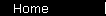### Sharpe Ratio

A number measuring the reward-to-risk efficiency of an investment, used to create risk-efficient portfolios.

The definition of the Sharpe Ratio is:

S(x) = (rx - Rf) / StdDev(x)
where
x is some investment
rx is the average annual rate of return of x
Rf is the best available rate of return of a "riskless" security (ie cash)
StdDev(x) is the standard deviation of rx